# Properties of Parallelograms

Go back to  'Triangles-Quadrilaterals'

The definition of a parallelogram is that it is a quadrilateral in which the opposite sides are parallel. From this definition, we will show that:

1. The opposite sides of a parallelogram are equal (and conversely: if the opposite sides of a quadrilateral are equal, it is a parallelogram).

2. The opposite angles of a parallelogram are equal (and conversely: if the opposite angles of a quadrilateral are equal, it is a parallelogram).

3. The diagonals of a parallelogram bisect each other (and conversely: if the diagonals of a quadrilateral bisect each other, it is a parallelogram).

4. If one pair of opposite sides of a quadrilateral is equal and parallel, then the quadrilateral is a parallelogram.

Theorem: In a parallelogram, opposite sides are equal. Conversely, if the opposite sides in a quadrilateral are equal, then it is a parallelogram.

Consider the following figure: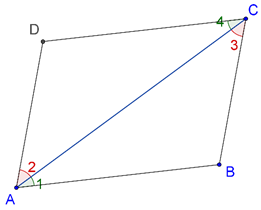Proof: First, we suppose that ABCD is a parallelogram. Compare $$\Delta ABC$$ and$$\Delta CDA$$:

1. AC = AC (common side)

2. $$\angle 1$$ = $$\angle 4$$ (alternate interior angles)

3. $$\angle 2$$ = $$\angle 3$$ (alternate interior angles)

Thus, by the ASA criterion, the two triangles are congruent, which means that the corresponding sides must be equal. Thus, AB = CD and AD = BC.

Now, we will prove the converse of this. Suppose that ABCD is a quadrilateral in which AB= CD and AD = BC. Compare $$\Delta ABC$$ and $$\Delta CDA$$ once again:

1. AC = AC (common side)

2. AB = CD (given)

3. AD = BC (given)

Thus, by the SSS criterion, the two triangles are congruent, which means that the corresponding angles are equal:

1. $$\angle 1$$ = $$\angle 4$$ è AB || CD

2. $$\angle 2$$ = $$\angle 3$$    è AD || BC

Theorem: In a parallelogram, opposite angles are equal. Conversely, if the opposite angles in a quadrilateral are equal, then it is a parallelogram.

Consider the following figure: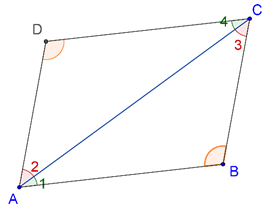Proof: First, we suppose that ABCD is a parallelogram. Compare $$\Delta ABC$$ and $$\Delta CDA$$ once again:

1. AC = AC (common side)

2. $$\angle 1$$ = $$\angle 4$$ (alternate interior angles)

3. $$\angle 2$$ = $$\angle 3$$ (alternate interior angles)

Thus, the two triangles are congruent, which means that $$\angle B$$ = $$\angle D$$.Similarly, we can show that $$\angle A$$ = $$\angle C$$. This proves that opposite angles in any parallelogram are equal.

Now, we prove the converse of this. Suppose that $$\angle A$$ = $$\angle C$$ and $$\angle B$$ = $$\angle D$$. We have to prove that ABCD is a parallelogram. Consider the following figure: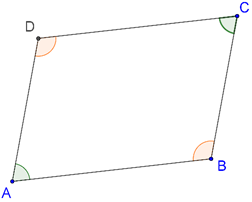We have:

$$\angle A$$ + $$\angle B$$ + $$\angle C$$ + $$\angle D$$ = 3600

è 2($$\angle A$$ + $$\angle B$$) = 3600

è$$\angle A$$ + $$\angle B$$ = 1800

This must mean that AD || BC (why?). Similarly, we can show that AB || CD, and thus, ABCD is a parallelogram.

Theorem: In a parallelogram, the diagonals bisect each other. Conversely, if the diagonals in a quadrilateral bisect each other, then it is a parallelogram.

Consider the following figure: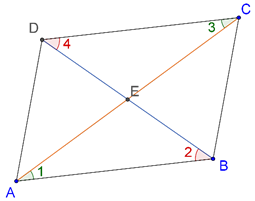Proof: First, let us suppose that ABCD is a parallelogram. Compare $$\Delta AEB$$ and $$\Delta DEC$$. We have:

1. AB = CD (opposite sides of a parallelogram)

2. $$\angle 1$$ = $$\angle 3$$ (opposite interior angles)

3. $$\angle 2$$ = $$\angle 4$$ (opposite interior angles)

By the ASA criterion, the two triangles are congruent, which means that AE = EC and BE =ED. Thus, the two diagonals bisect each other.

Now, we will prove the converse of this. Suppose that the diagonals AC and BD bisect each other. Compare $$\Delta AEB$$ and $$\Delta DEC$$ once again. We have:

1. AE = EC (given)

2. BE = ED (given)

3. $$\angle AEB$$ = $$\angle DEC$$ (vertically opposite angles)

By the SAS criterion, the two triangles are congruent, which means that:

1. $$\angle 1$$ = $$\angle 3$$è AB || CD

2. $$\angle 2$$ = $$\angle 4$$ è AD || BC

Thus, ABCD is a parallelogram.

Theorem: In a quadrilateral ABCD, if one pair of opposite sides is equal and parallel, then it is a parallelogram.

Consider the following figure: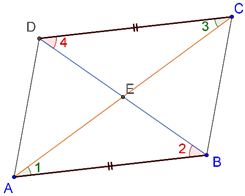It is given that AB = CD and AB || CD. We have to prove that ABCD is a parallelogram.

Proof: Compare $$\Delta AEB$$ and $$\Delta DEC$$. We have:

1. AB = CD (given)

2. $$\angle 1$$ = $$\angle 3$$ (opposite interior angles)

3. $$\angle 2$$ = $$\angle 4$$ (opposite interior angles)

Thus, the two triangles are congruent, which means that AE = EC and BE = ED. Therefore,the diagonals AC and BD bisect each other, and this further means that ABCD is a parallelogram (we have already proved this).

Let us summarize our discussion up to now. We have shown that the following statements are equivalent, that is, you can use them interchangeably:

1. A quadrilateral is a parallelogram

2. Opposite sides of a quadrilateral are equal

3. Opposite angles of a quadrilateral are equal

4. Diagonals of a quadrilateral bisect each other

5. One pair of opposite sides is equal and parallel

Example 1: If one angle of a parallelogram is 900, show that all its angles will be equal to 900.

Solution: In any parallelogram, the alternate angles are equal, and the adjacent angles are supplementary. Consider the parallelogram ABCD in the following figure, in which $$\angle A$$ is a right angle: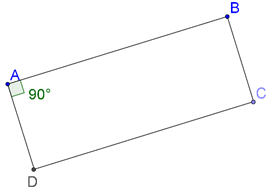Thus, $$\angle C$$ must also be 900, while

$$\angle B$$ = $$\angle D$$ = 1800 - 900 = 900

Clearly, all the angles in this parallelogram (which is actually a rectangle) are equal to 900.

Example 2:. In a quadrilateral ABCD, the diagonals AC and BD bisect each other at right angles. Show that the quadrilateral is a rhombus.

Solution: Consider the following figure: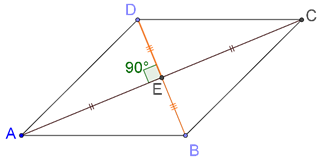First of all, we note that since the diagonals bisect each other, we can conclude that ABCD is a parallelogram. Now, let us compare $$\Delta AEB$$ and $$\Delta AED$$:

1. AE = AE (common)

2. BE = ED (given)

3. $$\angle AEB$$ = $$\angle AED$$ = 900 (given)

Thus, by the SAS criterion, the two triangles are congruent, which means that AB = AD.This further means that

AB = BC = CD = AD

Clearly, ABCD is a rhombus.

Example 3: ABCD is a quadrilateral in which the diagonals bisect each other. Show that B and D are equidistant from AC.

Solution: Since its diagonals bisect each other, ABCD is a parallelogram. Consider the following figure: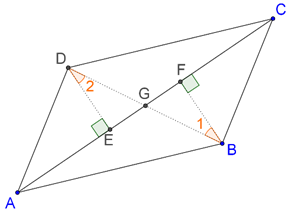Essentially, we have to show that BF = DE. Compare $$\Delta BFG$$ with $$\Delta DEG$$.

1. BG = GD (diagonals bisect each other)

2. $$\angle BGF$$ = $$\angle DGE$$ (vertically opposite angles)

3. $$\angle 1$$ = $$\angle 2$$ (alternate interior angles) (how?)

By the ASA criterion, the two triangles are congruent, which means that BF = DE. Thus, B and D are equidistant from A.

Example 4: If in a quadrilateral ABCD, AC bisects angles A and C, show that AC is perpendicular to BD.

Solution: Consider the following figure: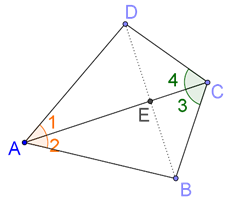Compare $$\Delta ABC$$ and $$\Delta ADC$$:

1. AC = AC (common)

2. $$\angle 1$$ = $$\angle 2$$ (given)

3. $$\angle 3$$ = $$\angle 4$$ (given)

By the ASA criterion, the two triangles are congruent. This means that AB = AD. Now,compare $$\Delta AEB$$ and $$\Delta AED$$:

1. AE = AE (common)

2. AB = AD (shown above)

3. $$\angle 2$$ = $$\angle 1$$

By the SAS criterion, the two triangles are congruent. Clearly,

$$\angle AEB$$ = $$\angle AED$$ = ½ × 1800 = 900

Example 5: Prove that the bisectors of the angles in a parallelogram form a rectangle.

Solution: Consider the following figure, in which ABCD is a parallelogram, and the dotted lines represent the (four) angle bisectors. We have to show that EFGH is a rectangle: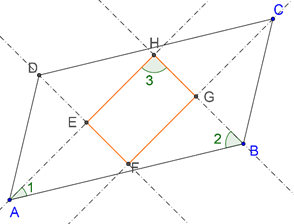We can show this by proving that each of the four angles of EFGH is a right angle. In parallelogram ABCD, we have

$$\angle A$$ + $$\angle B$$ = 1800

Now, consider $$\Delta AHB$$. We have:

$$\angle 1$$ + $$\angle 2$$ = ½ ($$\angle A$$ + $$\angle B$$) = 900

Thus, $$\angle 3$$ = 900. Similarly, we can prove that each of the other three angles of quadrilateral EFGH is a right angle. This means that EFGH is a rectangle.

Example 6: ABC is an isosceles triangle in which AB = AC. AD bisects exterior angle PAC and CD is parallel to AB, as shown below: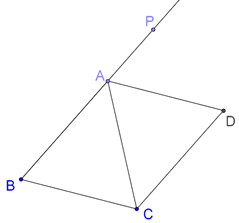Show that:

1. $$\angle DAC$$ = $$\angle BCA$$

2. ABCD is a parallelogram

Solution: We note that since $$\Delta ABC$$ is isosceles (with AB = AC), $$\angle B$$ =$$\angle BCA$$. Now, by the exterior angle theorem,

$$\angle PAC$$ = $$\angle BCA$$ + $$\angle B$$ = 2$$\angle BCA$$

è ½ $$\angle PAC$$ = $$\angle DAC$$ = $$\angle BCA$$

è BC || AD

Combining this with the fact given to us that CD || AB, we conclude that ABCD is a parallelogram.

Example 7: Two parallel lines are intersected by a transversal. Consider the quadrilateral ABCD formed by the bisectors of the interior angles, as shown below: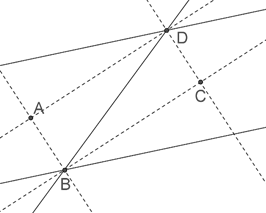Show that ABCD is a rectangle.

Solution: We first note that the two bisectors of any pair of lines are perpendicular to each other (if you are unsure about this, take a moment to prove it). Thus,

AB ⊥ BC and AD ⊥ DC.

Next, we note the following:

$$\angle ABD$$ + $$\angle ADB$$ = ½ (1800) = 900 (why?)

è $$\angle BAD$$ = 900

Similarly, $$\angle BCD$$ = 900. Thus, we have proved that all the four angles of the quadrilateral ABCD are right angles, which means that ABCD is a rectangle.